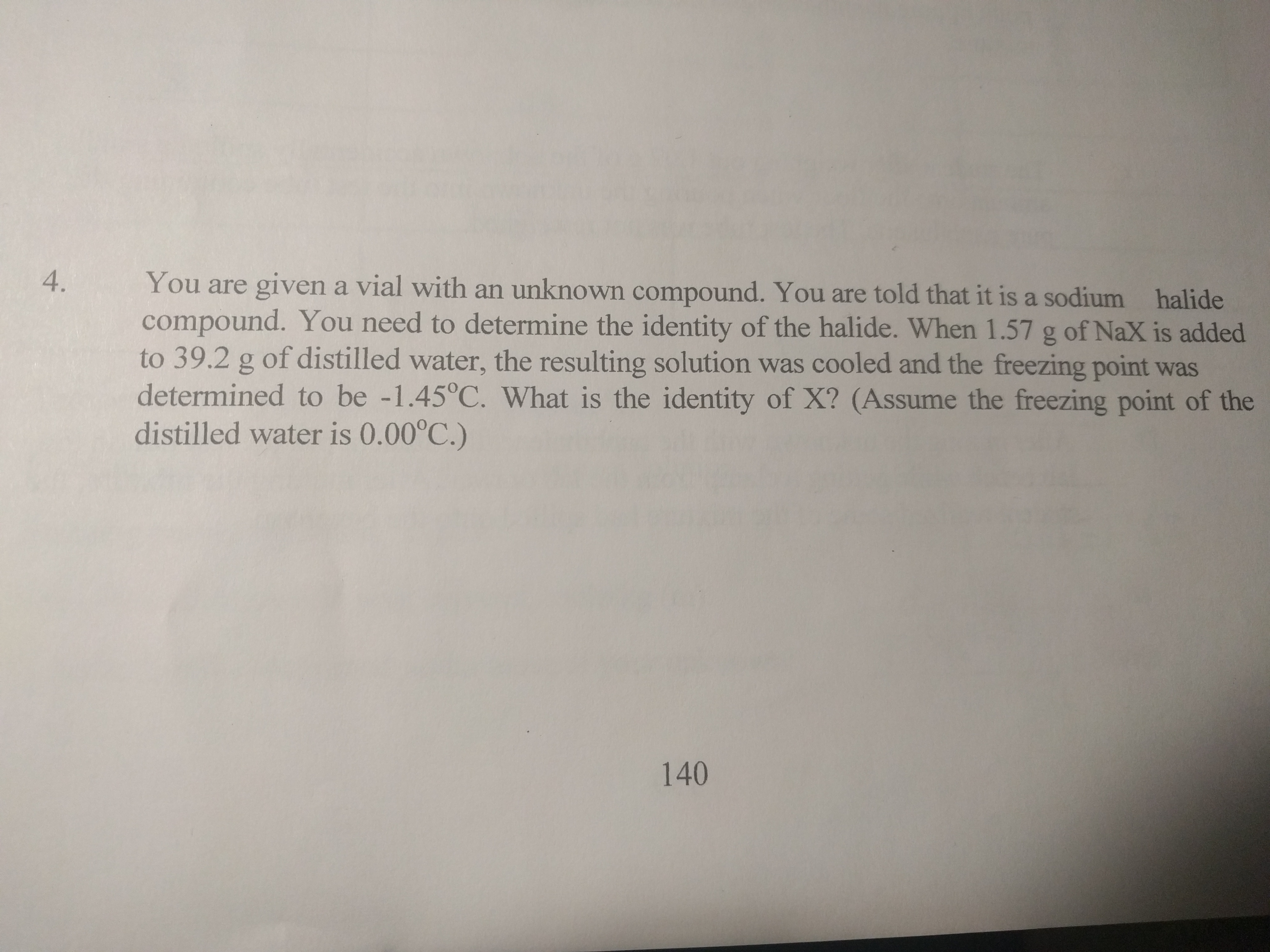You are given a vial with an unknown compound. You are told that it is a sodiumcompound. You need to determine the identity of the halide. When 1.57 g of NaX is addedto 39.2 g of distilled water, the resulting solution was cooled and the freezing point wasdetermined to be -1.45 °C. What is the identity of X? (Assume the freezing point of thedistilled water is 0.00°C.)4.halide140

Questionhelp_outlineImage TranscriptioncloseYou are given a vial with an unknown compound. You are told that it is a sodium compound. You need to determine the identity of the halide. When 1.57 g of NaX is added to 39.2 g of distilled water, the resulting solution was cooled and the freezing point was determined to be -1.45 °C. What is the identity of X? (Assume the freezing point of the distilled water is 0.00°C.) 4. halide 140 fullscreen
Step 1

Freezing point is a colligative property that means it depends upon the amount of solute or ions in solution.

Step 2

Given here,

mass of NaX = 1.57 g

dissolved in mass of water = 39.2 g = 0.0392 kg

[1 kg = 1000 g]

freezing point of solution = -1.45 degree C

Depression in freezing point (dTf) = -1.45 - 0 = -1.45 degree C

Kf for water = 1.86 degree C/m

i (Van't hoff factor) = 2 [NaX has 2 ions Na+ and X-]

Using,

dTf = -i x Kf x m

-1.45 = -2 x 1.86 x m

molality of solution (m) = 0.39 m

with,

molality = mass of solute/kg of solvent

we get,

moles of NaX = 0.39 m x 0.0392 kg

...

Want to see the full answer?

See Solution

Want to see this answer and more?

Our solutions are written by experts, many with advanced degrees, and available 24/7

See Solution
Tagged in

Solutions